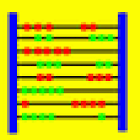Abakus-Equa

All Android applications categories

All Android games categories# Abakus-Equa

by: 0 0

## Screenshots

Description

Abakus-Equa is a computer algebra program which performs
symbolic and numerical calculations on mathematical equations.

The current Android version 1.0 contains the following functions:
- Exact solution of linear and non-linear equations
- Numerical solution of linear and non-linear equations
- Exact solution of systems of linear equations

For example, the input "ln(x+4) = 0" with variable "x" yields the output "x = -3".
The equation "7*cosh(x) = sinh(x)+9" resolves to "x = ln((9-sqrt(33))/6); x = ln((9+sqrt(33))/6)".
For the system of equations "x+2*y = 3; 4*x+5*y = 7",
Abakus-Equa calculates the exact solution "x = -1/3; y = 5/3".
The variable system "x+a*y = 2; x-y = 0" resolves to "x = y = 2/(1+a)".

Moreover Abakus-Equa contains a special editor for mathematical formulas.
So you can enter even complex expressions very fast.

Tags: abakusequa , abaküs